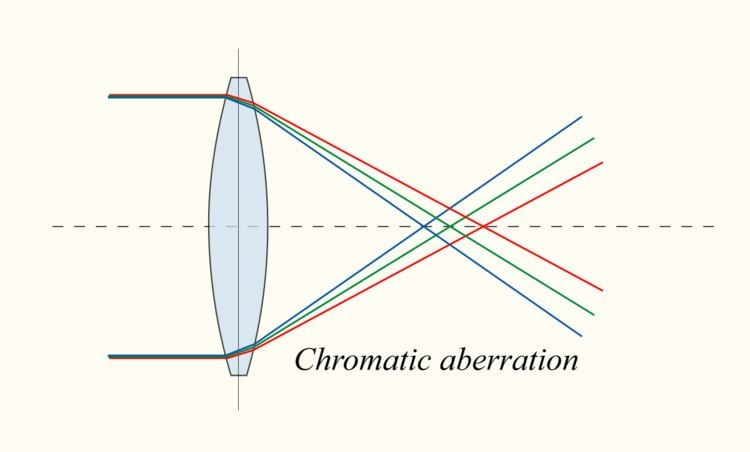# Achromatic lens

Updated on
Covid-19An achromatic lens or achromat is a lens that is designed to limit the effects of chromatic and spherical aberration. Achromatic lenses are corrected to bring two wavelengths (typically red and blue) into focus in the same plane.

## Contents

The most common type of achromat is the achromatic doublet, which is composed of two individual lenses made from glasses with different amounts of dispersion. Typically, one element is a negative (concave) element made out of flint glass such as F2, which has relatively high dispersion, and the other is a positive (convex) element made of crown glass such as BK7, which has lower dispersion. The lens elements are mounted next to each other, often cemented together, and shaped so that the chromatic aberration of one is counterbalanced by that of the other.

In the most common type (shown), the positive power of the crown lens element is not quite equalled by the negative power of the flint lens element. Together they form a weak positive lens that will bring two different wavelengths of light to a common focus. Negative doublets, in which the negative-power element predominates, are also made.

## History

Theoretical considerations of the feasibility of correcting chromatic aberration were debated in the 18th century following Newton's statement that such a correction was impossible (see History of the telescope). Credit for the invention of the first achromatic doublet is often given to an English barrister and amateur optician named Chester Moore Hall. Hall wished to keep his work on the achromatic lenses a secret and contracted the manufacture of the crown and flint lenses to two different opticians, Edward Scarlett and James Mann. They in turn sub-contracted the work to the same person, George Bass. He realized the two components were for the same client and, after fitting the two parts together, noted the achromatic properties. Hall failed to appreciate the importance of his invention, and it remained known to only a few opticians.

In the late 1750s, Bass mentioned Hall's lenses to John Dollond, who understood their potential and was able to reproduce their design. Dollond applied for and was granted a patent on the technology in 1758, which led to bitter fights with other opticians over the right to make and sell achromatic doublets.

Dollond's son Peter invented the apochromat, an improvement on the achromat, in 1763.

## Types

Several different types of achromat have been devised. They differ in the shape of the included lens elements as well as in the optical properties of their glass (most notably in their optical dispersion or Abbe number).

In the following, 'R' denotes the radius of the spheres that define the optically relevant refracting lens surfaces. By convention, R1 denotes the first lens surface counted from the object. A doublet lens has four surfaces with radii R1 to R4.

## Littrow doublet

Uses an equiconvex crown glass lens with R1=R2, and a second flint glass lens with R3=-R2. The back of the flint glass lens is flat. A Littrow doublet can produce a ghost image between R2 and R3 because the lens surfaces of the two lenses have the same radii. When used in a telescope, it may also produce a ghost image between the flat R4 surface and rear of the tube.

## Fraunhofer doublet (Fraunhofer objective)

The first lens has positive refractive power, the second negative. R1 is set greater than R2, and R2 is set close to, but not equal to, R3. R4 is usually greater than R3. In a Fraunhofer doublet, the dissimilar curvatures of R2 and R3 are mounted close, but not in contact. This design yields more degrees of freedom (one more free radius, length of the air space) to correct for optical aberrations.

## Clark doublet

Uses an equiconvex crown with R1=R2, and a flint with R3≃R2 and R4≫R3. R3 is set slightly shorter than R2 to create a focus mismatch between R2 and R3, thereby reducing ghosting between the crown and flint.

## Oil-spaced doublet

The use of oil between the crown and flint eliminates the effect of ghosting, particularly where R2=R3. It can also increase light transmission slightly and reduce the impact of errors in R2 and R3.

## Steinheil doublet

The Steinheil doublet, devised by Carl August von Steinheil, is a flint-first doublet. In contrast to the Fraunhofer doublet, it has a negative lens first followed by a positive lens. It needs stronger curvature than the Fraunhofer doublet.

## Dialyte

Dialyte lenses have a wide air space between the two elements. They were originally devised in the 19th century to allow much smaller flint glass elements down stream since flint glass was hard to produce and expensive. They are also lenses where R2 and R3 can not be cemented because they have dissimilar curvatures.

## Design

The first-order design of an achromat involves choosing the overall power ϕ sys of the doublet and the two glasses to use. The choice of glass gives the mean refractive index, often written as n d (for the refractive index at the Fraunhofer "d" spectral line wavelength), and the Abbe number V (for the reciprocal of the glass dispersion). To make the linear dispersion of the system zero, the system must satisfy the equations

ϕ 1 + ϕ 2 = ϕ sys ϕ 1 V 1 + ϕ 2 V 2 = 0   ,

where the lens power is ϕ = 1 / f for a lens with focal length f . Solving these two equations for ϕ 1 and ϕ 2 gives

ϕ 1 ϕ sys = V 1 V 1 V 2 and ϕ 2 ϕ sys = V 2 V 1 V 2   .

Since ϕ 2 = ϕ 1 V 2 / V 1 , and the Abbe numbers are positive-valued, the power of the second element in the doublet is negative when the first element is positive.

Topics